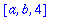Normally, when you enter an expression it is evaluated completely. If your expression contains a variable that has a value, the variable is replaced by that value; if it contains a function call such as f(x) , where f is a procedure or function, then this is replaced by the value returned by f with argument x . And if those values in turn contain variables or function calls, they are also evaluated. Eventually (we hope) Maple obtains a form that does not require any further evaluation, and this is what it returns to us. However, certain types of object have special evaluation rules. In particular, tables, arrays (including vectors and matrices) and procedures use last name evaluation. This means that if the result of one level of evaluation of a name would be a table, array or procedure, then the chain of evaluation stops with that name. For example:

> T:= array([a,b,c]);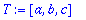> S:= T;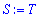> S;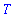In the first statement, T is assigned a value which is an array. Then S is assigned the value T . Evaluation of T stops at the name, rather than going all the way to the array [1,2,3] . For S there is one level of evaluation, yielding T , but again evaluation stops there. If you want the next level of evaluation, the table or array structure, you can use eval :

> eval(S);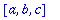However, even this is not full evaluation, as the entries of this array were not evaluated. For that you need to map eval into S :

> b:= a: a:= sin(x): x:= Pi:

> eval(S);> map(eval,S);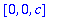Last-name evaluation is usually not a problem, and can have significant benefits in efficiency by avoiding the copying of large tables or arrays. However, there are some points to watch out for. One is that at first glance it may appear that T has not been assigned a value. Even the whattype function does not help here:

> whattype(T);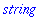Instead, you can use assigned . Of course you could also use eval(T) .

> assigned(T);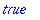> whattype(eval(T));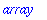Another common problem is that subs will not see the table, array or procedure structure, but only the name. Again, you can fix this by using eval .

> subs(c=4, S);> subs(c=4, eval(S));